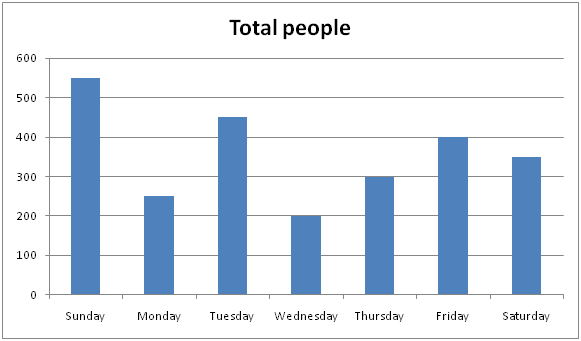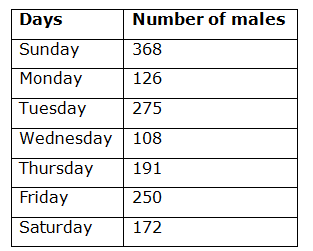# NIACL AO Mains– Quantitative Aptitude Questions Day- 26

Dear Readers, Bank Exam Race for the Year 2019 is already started, To enrich your preparation here we have providing new series of Practice Questions on Quantitative Aptitude – Section. Candidates those who are preparing for NIACL AO Mains 2019 Exams can practice these questions daily and make your preparation effective.

[WpProQuiz 5361]

1) A jar contains 85 litres of mixture of milk and water in the ratio of 15: 2. 51 litres of mixture is taken out and 2 litres of water is added to the jar. What is the percentage of water in the mixture?

a) 20 %

b) 25 %

c) 16 (2/3) %

d) 28 (1/3) %

e) None of these

2) The sum of two numbers is 10620. 45 % of one number is equal to 36 % of another number. Find the difference between those two numbers

a) 1180

b) 1260

c) 1320

d) 1450

e) None of these

3) The marked price of A is Rs. 2400 more than the cost price. When the discount of 500 is allowed, the profit of 20% is earned. At what price should A be sold to earn the profit of 30%?

a) Rs. 16755

b) Rs. 12350

c) Rs. 11960

d) Rs. 13845

e) None of these

4) A, B and C started a work together for Rs. 3230. A and B did 13/19 of the total work, while B and C did 11/19 of the whole work. Find the wage of the least efficient person?

a) Rs. 800

b) Rs. 900

c) Rs. 950

d) Rs. 850

e) None of these

5) The ratio of the present ages of Priya to that of Moni is 3: 4. Priya is 6 years older than Revathi, who is 14 years younger than Moni. Find the present age of Revathi

a) 24 years

b) 20 years

c) 22 years

d) 18 years

e) None of these

Directions (Q. 6 – 10): Study the following information carefully and answer the given questions:

The following bar graph shows the total number of people went for a movie in different days of a week in a certain village and the table shows the total number of males among them.6) Total number of females went for a movie in Tuesday and Friday together is approximately what percentage of total number of males went for a movie in Monday and Wednesday together?

a) 120 %

b) 105 %

c) 140 %

d) 160 %

e) 90 %

7) Find the ratio between the total number of males went for a movie in Sunday and Saturday together to that of total number of females went for a movie in Wednesday and Thursday together?

a) 180: 67

b) 152: 53

c) 217: 93

d) 375: 158

e) None of these

8) If 34 % of total number of people went for a movie in Sunday bought AC tickets, then find the difference between total number of people buy AC tickets in Sunday to that of total number of females went for a movie in Monday?

a) 82

b) 56

c) 75

d) 63

e) None of these

9) In which of the following days, the lowest number of females went for a movie?

a) Thursday

b) Wednesday

c) Monday

d) Tuesday

e) None of these

10) Find the average number of males went for a movie in Monday to Friday?

a) 210

b) 170

c) 190

d) 180

e) None of these

Quantity of water in the mixture 51 litres = 51 * (2/17) = 6 litres

Quantity of milk in the mixture 51 litres = 51 * (15/17) = 45 litres

Remaining mixture = 85 – 51 = 34 litres

Now, 2 litres of water is added,

= > 34 *(2/17) + 2 = 4 + 2 = 6 litres

Percentage of water = (6/36)*100 = 200/9 = 16 (2/3) %

Let the two numbers be x and y,

X + y = 10620

(45/100)*x = (36/100)*y

(X/y) = 4/5

9’s = 10620

1’s = 10620/9 = 1180

Required difference = (5’s – 4’s) = 1’s = 1180

Let the C.P be x,

M.P = 2400 + C.P = 2400 + x

1. P = (2400 + x) – 500 = 1900 + x

S.P = (120/100)*x = (6x/5)

1900 + x = 6x/5

9500 + 5x = 6x

C.P (x) = 9500

To earn a profit of 35 %,

1. P = (130/100)*9500 = Rs. 12350

(A+B)’s work = 13/19

(B+C)’s work = 11/19

Now, (A+B+B+C) – (A+B+C) = B

13/19 + 11/19 – 1 = B

B = (24/19) – 1 = 5/19

A + B = 13/19

A = 13/19 – 5/19 = 8/19

B + C = 11/19

C = 11/19 – 5/19 = 6/19

So, Ratio of distribution = (8/19): (5/19): (6/19) = 8: 5: 6

B is the least efficient person

So, B’s share = (5/19)*3230 = Rs. 850

Let the present age of Moni be x,

Revathi’s present age = Moni – 14 = x – 14

Priya’s present age = 6 + Revathi’s present age = 6 + x – 14 = x – 8

According to the question,

(X – 8) / x = ¾

4x – 32 = 3x

X = 32

Revathi’s present age = 32 – 14 = 18 years

Direction (6-10) :

Total number of females went for a movie in Tuesday and Friday together

= > (450 – 275) + (400 – 250)

= > 175 + 150 = 325

Total number of males went for a movie in Monday and Wednesday together

= > 126 + 108 = 234

Required % = (325/234)*100 = 138. 889 % = 140 %

The total number of males went for a movie in Sunday and Saturday together

= > 368 + 172 = 540

The total number of females went for a movie in Thursday and Wednesday together

= > (300 – 191) + (200 – 108)

= > 109 + 92 = 201

Required ratio = 540: 201 = 180: 67

34 % of total number of people went for a movie in Sunday bought AC tickets

= > 550*(34/100) = 187

Total number of females went for a movie in Monday

= > 250 – 126 = 124

Required difference = 187 – 124 = 63

In Sunday = > 550 – 368 = 182

In Monday = > 250 – 126 = 124

In Tuesday = > 450 – 275 =175

In Wednesday = > 200 – 108 = 92

In Thursday = > 300 – 191 = 109

In Friday = > 400 – 250 = 150

In Saturday = > 350 – 172 = 178

The lowest number of females went for a movie in Wednesday.